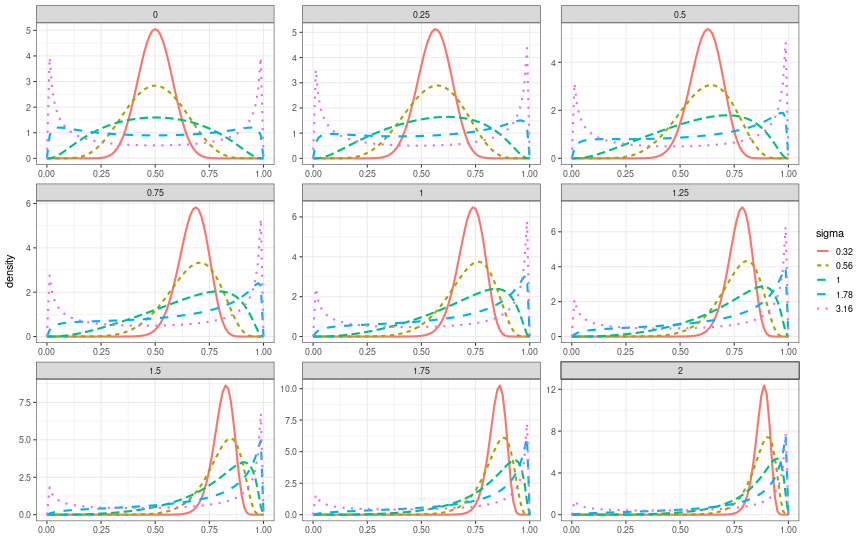# Basic usage

## Distribution

The logitnormal distribution is useful as a prior density for variables that are bounded between 0 and 1, such as proportions. The following figure displays its density for various combinations of parameters mu (panels) and sigma (lines).Example: Plot the cumulative distribution

x <- seq(0,1, length.out=81)
d <- plogitnorm(x, mu=0.5, sigma=0.5)
plot(d~x,type="l")## Mean and Variance

The moments have no analytical solution. This package estimates them by numerical integration:

Example: estimate mean and standard deviation.

(theta <- momentsLogitnorm(mu=0.6,sigma=0.5))

##       mean        var
## 0.63812093 0.01208171


## Mode

The mode is found by setting derivatives to zero and optimizing the resulting equation: $$logit(x) = \sigma^2(2x-1)+\mu$$.

Example: estimate the mode

(mle <- modeLogitnorm(mu=0.6,sigma=0.5))

##  0.6641416


## Parameter Estimation

from upper quantile and

• mode (Maximum Likelihood Estimate)
• mean (Expected value)
• median

Example: estimate the parameters, with mode 0.7 and upper quantile 0.9

(theta <- twCoefLogitnormMLE(0.7,0.9))

##             mu    sigma
## [1,] 0.7608886 0.464783

x <- seq(0,1, length.out=81)
d <- dlogitnorm(x, mu=theta[1,"mu"], sigma=theta[1,"sigma"])
plot(d~x,type="l")
abline(v=c(0.7,0.9), col="grey")When increasing the $$\sigma$$ parameter, the distribution becomes eventually becomes bi-model, i.e. has two maxima. The unimodal distribution for a given mode with widest confidence intervals is obtained by function twCoefLogitnormMLEFlat.

(theta <- twCoefLogitnormMLEFlat(0.7))

##             mu    sigma
## [1,] 0.0335529 1.426311

x <- seq(0,1, length.out=81)
d <- dlogitnorm(x, mu=theta[1,"mu"], sigma=theta[1,"sigma"])
plot(d~x,type="l")
abline(v=c(0.7), col="grey")